Shallow Foundations - 1

# Shallow Foundations - 1 - Notes | Study Foundation Engineering - Civil Engineering (CE)

 Table of contentsShallow Foundation & Bearing Capacityor1 Crore+ students have signed up on EduRev. Have you?

Shallow Foundation & Bearing Capacity

Bearing Capacity

It is the load carrying capacity of the soil.

1. Ultimate bearing capacity or Gross bearing capacity (qu)
It is the least gross pressure which will cause shear failure of the supporting soil immediately below the footing.
2. Net ultimate bearing capacity (qun)
It is the net pressure that can be applied to the footing by external loads that will just initiate failure in the underlying soil. It is equal to ultimate bearing capacity minus the stress due to the weight of the footing and any soil or surcharge directly above it. Assuming the density of the footing (concrete) and soil (γ) are close enough to be considered equal, then
qnu = qu - γDy
Where, Df is the depth of footing
3. Safe bearing capacity
It is the bearing capacity after applying the factor of safety (FS). These are of two types,

Safe net bearing capacity (qns)
It is the net soil pressure which can be safety applied to the soil considering only shear failure. It is given by,
qNS = qn2/FS

Safe gross bearing capacity (qs)
It is the maximum gross pressure which the soil can carry safely without shear failure. It is given by,
qs = qns + γDf

Allowable Bearing Pressure
It is the maximum soil pressure without any shear failure or settlement failure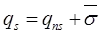where, qs = Safe bearing capacity.

Method to determine bearing capacity

1. Rankines Method (∅ - soil)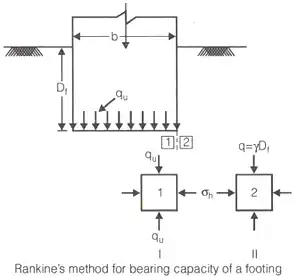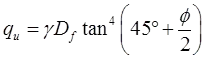or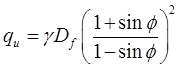2. Bells Theory (C - ∅)
qu = CNc + γDfNq
where, Nc and Nq are bearing capacity factors.
For pure clays → C = 4, q = 1
3. Fellinious Method: (C-soil)
(i) The failure is assumed to take place by slip and the consequent heaving of a mass of soil is on one side.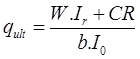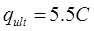(ii) Location of Critical circle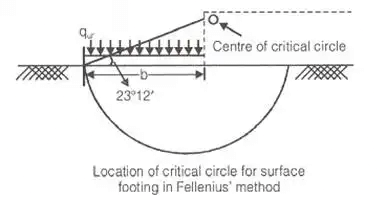4. Prandtl Method: (C - ∅)
(i) For strip footing

qu = CNc + γDfNq + 1/2γBNγ
(ii) For C-soil
Nc = 5.14, Nq = 1, Nγ = 0
5. Terzaghi Method (C - ∅)
(i) Assumptions
S – Strip footing, S – Shallow foundation, G – General shear failure, H – Horizontal ground, R – Rough base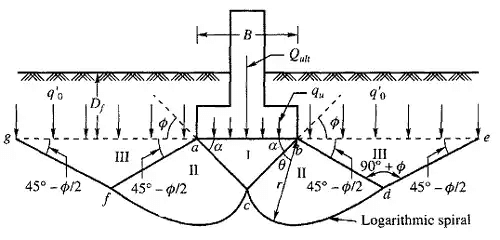(a) For strip footing
qu = CNc + γDfNq + (1/2) γBNγ
(b) For square footing
qu = 1.3CNc + γDfNq + 0.4 γBNγ
(c) For rectangular footing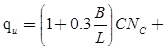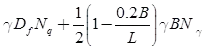(d) For circular footing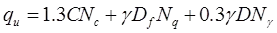where,
D = Dia of circular footing
CNc → Contribution due to constant component of shear strength of soil.
γDfN→ Contribution due to surcharge above the footing
1/2(γBNγ) → Contribution due to bearing capacity due to self weight of soil.
(ii) Bearing capacity factors
Nq = N∅.θπtan∅
where, N = influence factor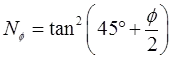Nγ = 1.8tan∅(Nq - 1)
NC = cot∅(Nq - 1)
For C-soil:
NC = 5.7, Nq = 1, Nγ = 0
6. Skemptons Method (c-soil)
This method gives net ultimate value of bearing capacity.
Applicable for purely cohesive soils only.
qnu = CNc
For strip footing.
NC = 5 to 7.5
For circular and square footing.
NC = 6 to 9.0
(i) Values of NC
(a) Df/B = 0 i.e.at the surface.
Then NC = 5 For strip footing
NC = 6.0 For square and circular footing.
where Df = Depth of foundation.
(b) If 0 ≤ Df/B ≤ 2.5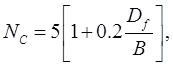for strip footing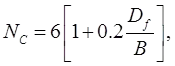For square and circular footing.
B = D in case of circular footing.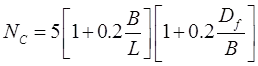for rectangular footing
(c) if Df/B ≤ 2.5 NC =7.5
for strip footing
NC = 9.0 for circular, square and rectangular footing.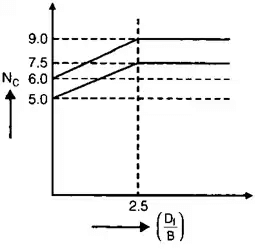7. Meyorhoff's Method → (C - ∅ soil)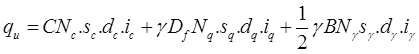8. IS code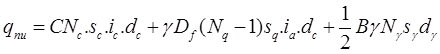The document Shallow Foundations - 1 - Notes | Study Foundation Engineering - Civil Engineering (CE) is a part of the Civil Engineering (CE) Course Foundation Engineering.
All you need of Civil Engineering (CE) at this link: Civil Engineering (CE)

## Foundation Engineering

18 videos|27 docs|20 tests
 Use Code STAYHOME200 and get INR 200 additional OFF

## Foundation Engineering

18 videos|27 docs|20 tests

Track your progress, build streaks, highlight & save important lessons and more!

,

,

,

,

,

,

,

,

,

,

,

,

,

,

,

,

,

,

,

,

,

;